# Kerala Syllabus 10th Standard Maths Solutions Chapter 10 Polynomials

## Kerala State Syllabus 10th Standard Maths Solutions Chapter 10 Polynomials

### Polynomials Textbook Questions & Answers

Textbook Page No. 237

Free Degree and Leading Coefficient Calculator – Find the leading coefficient of a polynomial function step-by-step.

Polynomials Class 10 Kerala Syllabus  Questions 1.
Write the second degree polynomials. given below as the product of two first degree polynomials. Find also the solutions of the equation p(x) = 0 in each.
i. p(x) = x2 – 7x+12
ii. p(x) = x2 + 7x + 12
iii.p (x) = x2 – 8x +12
iv. p(x) = x2 + 13x +12
v. p (x) = x2 + 12x – 13
vi. p (x) = x2 – 12x – 13
i. p (x) = x2 – 7x + 12
a + b = –7, ab = 12
a = –3, b = –4
x2 – 7x + 12 = (x – 3) (x – 4)
x2 – 7x + 12 = 0
(x – 3) (x – 4) = 0
x – 3 = 0, x – 4 = 0
x = 3, x = 4
ii. p(x) = x2 + 7x + 12
a + b = 7, ab= 12 a = 3, b = 4
x2 + 7x + 12 = (x + 3) (x + 4)
x2 + 7x + 12 = 0
(x + 3)(x + 4) = 0
x = 3, x = 4
iii. p(x) = x2 – 8x + 12
a + b = 8, ab= 12
a = 6, b = –2
x2 – 8x + 12 = (x – 6) (x – 2)
(x – 6) (x – 2) = 0
x = 6, x = 2
iv. p(x) =x2 + 13x + 12
a+b = 13, ab = 12
a =12, b=1
(x2 +13x + 12) = (x + 12) (x + 1)
x2+13x + 12 = 0
(x+ 12) (x+ 1) = 0
x+ 12 = 0, x+ 1 = 0
x = -12 ,x = –1
v. p(x) = x2 + 12x – 13
a = –13, b = 1
x2 + 12x – 13 = (x + 13)(x – 1)
x + 13 = 0, x – 1 =0
x = –13, x= 1 .
vi. p(x) = x2 – 12x – 13
x2 – 12x – 13 = (x – a) (x – b)
= x2 – (a + b) x + ab
a + b= 12 ab = –13
(a – b)2 =(a + b)2 – 4ab
= (12)2 – 4x – 13 = 196
a – b = 14
a + b= 12
a= 13; b = –1
x2 – 12x – 13 = (x – 13)(x + 1)
x – 13 = 0, x + 1 =0
x= 13 ,x = –1Textbook Page No. 240

Is this a Polynomial Calculator is an online tool that helps to calculate the result of addition, subtraction, multiplication, and division of two polynomials.

Sslc Maths Chapter 10 Kerala Syllabus Questions 1.
In each pair of polynomials given below, find the number to be subtracted from the first to get a polynomial for which the second is as factor. Find also the second factor of the polynomial got on subtracting the number.
i. x2 – 3x + 5, x – 4
ii. x2 – 3x + 5, x + 4
iii. x2 + 5x – 7, x – 1
iv. x2 – 4x – 3, x – 1
p(x) = x2 – 3x + 5
If x – 4 is a factor, p(4) = 0
p(4) = (4)2 – 3 x 4 + 5 = 9
For x – 4 to become a factor of p (4) must be equal to zero.
For p (4) = 0 here we have to subtract 9 from p(x).
That is, add -9 to p(x) for (x – 4) become a factor.
∴ p(x) = x2 – 3x + 5 – 9 = x2 – 3x – 4
x2 – 3x – 4 = (x – a) (x – b)
= x2 – (a + b) x + ab
a + b = 3
ab = –4
(a – b)2 = (a + b)2 – 4 ab
= (3)2 – 4x – 4 = 25
a – b = 5
a + b = 3
a = 4; b = –1
x2 – 3x – 4 = (x – 4)(x + 1)
Second factor is (x +1)
ii. p(x) = x2 – 3x + 5
If x + 4 is a factor, p(–4) = 0
p(–4) = (–4)2 – 3x – 4 + 5 = 33
For x + 4 to become a factor of p (–4)
must be equal to zero.
For p (–4) = 0 here we have to subtract 33 from p(x).
That is, add –33 to p(x) for (x + 4) become a factor.
∴p(x) = x2 – 3x + 5 – 33 = x2 – 3x – 28
x2 – 3x – 28 = (x – a) (x – b)
= x2 – (a+b) x + ab
a + b = 3
ab = -28
(a – b)2 = (a + b)2 – 4ab
= (3)2 – 4x – 28 = 121
a – b= 11
a + b = 3
a = 7;
b = -4 x2 – 3x – 28 = (x – 7)(x + 4)
Second factor is (x – 7)
iii. p(x) = x2 + 5x – 7
If x – 1 is a factor, p(1) = 0
p(1) = (1)2 +5 x 1 – 7 = –1
For x – 1 to become a factor of p (1) must be equal to zero.
For p (1) = 0 here we have to subtract –1 from p(x).
That is, add 1 to p(x) for (x – 1) become a factor.
p(x) = x2 + 5x – 7 + 1 = x2 + 5x – 6
x2 + 5x – 6 = (x – a) (x – b)
a + b = 5
ab = –6
a = –6; b= 1
x2 + 5x – 6 = (x + 6)(x – 1)
Second factor is (x + 6)
iv. p(x) = x2 – 4x – 3
If x – 1 is a factor, p(1) = 0
p(1) = (1)2 – 4 x 1 – 3 = –6
For x – 1 to become a factor of p (1) must
be equal to zero.
For p (1) = 0 here we have to subtract -6 from p(x).
That is, add 6 to p(x) for (x – 1) become a factor.
∴ p(x) = x2 – 4 x – 3 + 6 = x2 – 4x +3
x2 – 4x + 3 = (x – a) (x – b)
a + b = –4 ab = 3
a =1; b = 3
x2 – 4x +3 = (x – 1)(x – 3)
Second factor is (x – 3)

Still, using a computation tool like a factor complex polynomials calculator, you can streamline things for you.

Polynomials Class 10 State Syllabus Questions 2.
In the polynomial x2 + kx + 6, what number must be taken ask to get a polynomial for which x –1 is a factor? Find also the other factor of that polynomial.
p (x) = x2 + kx + 6
If (x – 1) is a factor of p(x)
then p(1) = 0
p(1) = 12 + k x 1 + 6 = 7 + k
7 + k = 0
k= –7
∴ p(x) = x2 – 7x + 6
a + b = 7
ab = 6
a= 1, b = 6
factors are (x – 1 )(x – 6)
Second factor is (x – 6)

Polynomials Class 10 Kerala Syllabus Questions 3.
In the polynomial kx2 + 2x – 5, what number must be taken ask to get a polynomial for which x –1 is a factor?
p(x) = kx2 + 2x – 5
If (x – 1) is a factor, then p(1) = 0
p(1) = k(l)2 + 2 x 1 – 5
= k + 2 – 5 = k – 3
k – 3 = 0 k = 3

Textbook Page No. 242

Sslc Polynomials Questions And Answers Kerala Syllabus Question 1.
Write the second degree polynomials given below as die product of two first degree polynomials:
i. x2 – 20x + 91
ii. x2 – 20x + 51
iii. x2 + 5x – 84
iv. 4x2 – 16x +15
v. x2 – x – 1
p(x) = x2 – 20x + 91
We must solve the equation p(x) = 0
x2 – 20x + 91 = 0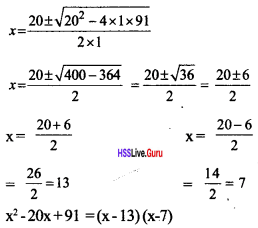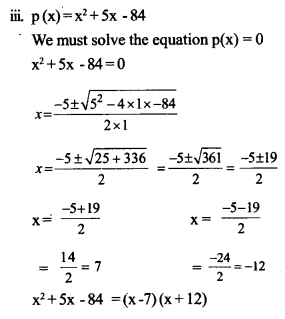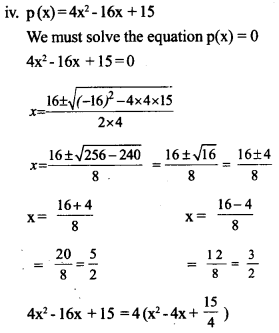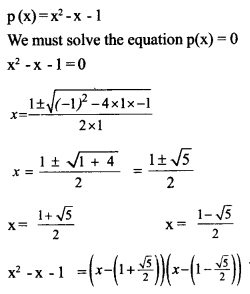Sslc Maths Polynomials Solutions Kerala Syllabus Question 2.
Prove that none of the polynomials below can be factored into a product of first degree polynomials:
i. x2 + x + l
ii. x2 – x + l
iii. x2 + 2x + 2
iv. x2 + 4x + 5
i. x2 + x+1
We must solve the equation p(x) = 0
x2 + x +1 =0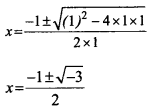no solutions
p(x) doesn’t have any first degree factors.
ii. x2 – x + 1
We must solve the equation p(x) = 0
x2 – x + 1 = 0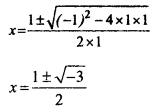no solutions
p(x) doesn’t have any first degree factors
iii. x2 + 2x + 2
We must solve the equation p(x) = 0
x2 + 2x + 2 = 0no solutions
p(x) doesn’t have any first degree factors.
iv. x2 + 4x + 5
We must solve the equation p(x) = 0
x2 + 4x + 5 = 0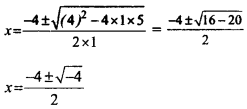no solutions
p(x) doesn’t have any first degree factors.

Sslc Maths Chapter 10 Solutions Kerala Syllabus Question 3.
In the polynomial p(x) = x2 + 4x + k, up to what number can we take ask, so that p(x) can be factorized as a product of two first degree polynomials?
p(x) = x2 + 4x + k
p(x) = 0
x2 + 4x + k = 0p(x) can be factorized as a product of two first degree polynomials, the $$\sqrt{16-4 k}>0$$ So, k can take value up to 4.

### Polynomials Orukkam Questions & Answers

Worksheet 1

Polynomials Class 10 Hsslive Kerala Syllabus Question 1.
Write the product (x – 1) x (x + 1)
Find the product of (x – 1),(x + 1),(x + 2) If the poduct is p(x)find p(1), (-1), p(-2) Write the solution of the equation p(x) = 0.
(x – 1)x (x + 1) = x2 – 1
(x – 1) (x + 1) (x + 2)=(x2 – 1) (x + 2)
= x3 – x + 2x2 – 2 = x3 + 2x2 – x – 2 = 0
p(x) = x3 + 2x2 – x – 2 = 0
p(1) =1 + 2 – 1 –  2 = 0
p(–1) = – 1 + 2 + 1 – 2 = 0
p(–2) = – 8 + 8 + 2 – 2 = 0
1, – 1, – 2 are the solutions of the equation p(x) = 0.Hss Live Guru 10th Maths Kerala Syllabus Question 2.
Expand (x – a)(x – b). If x2 – 7x + 12 = (x – a)(x – b) then find a+b.
Also find ab Calculate the values of a, b. Write the factors of (x2 – 7x +12 ).
Find the solutions of (x2 – 7x + 12).
(x – a) (x – b) = x2 – bx – ax + ab
= x2 – x (a + b) + ab
= x2 – 7x + 12 = (x – a)(x – b)
a + b = 7
ab = 12
(a + b)2 – 4ab = 72 – 4 x 12 = 49 – 48 = 1 = a – b
a + b = 7
a – b = 1, 2a = 8 a = $$\frac { 8 }{ 2 }$$ =4
b = 7 – 4 = 3 a = 4 and b = 3.
Solution of x2 – 7x + 12 is x2 – 7x + 12 = (x – 4) (x – 3)
Solutions = 4, 3

Maths Questions And Answers For Class 10 Kerala Syllabus Question 3.
If p(x) = x3 – 6×2 + 11x – 1 then find p(1), p(2), p(3). Find p(x) – p(1), p(x) – p(2), p(x) – p(3), p(x) – p(1). Write the solutions of p(x) – p(1) = 0
Polynomials Class 10 Worksheet with Answer:
p(x) = x3 – 6x2 + 11x – 1
p(1) = 1 – 6 + 11 – 1 = 5
p(2) = 8 – 24 + 22 – 1 = 5
p(3) = 27 – 54 + 33 – 1 = 5
p(x) – p(1) = x3 – 6x2 + 11x – 6
p(x) – p(2) = x3 – 6x2 + 11x – 6
p(x) – p(3) = x3 – 6x2 + 11x – 6
p(x) – p(1) = x3 – 6x2 + 11x – 6 = 0
If x = 1, 2, 3 then p(x) – p(1) = 0.
Factors of the equations are (x – 1), (x – 2), (x – 3).
Solutions of the equations are 1, 2, 3.

Hss Live Maths 10th Kerala Syllabus Question 4.
When p(x)is divided by (ax + b), the quotient is q(x)and the remainder is c. p(x) = (ax + b) x q(x) + c
When does the value of p(x) equal to c $$p\left(\frac{-b}{a}\right)=\left(a \times \frac{-b}{a}+b\right) \times q\left(\frac{-b}{a}\right)+c$$
What is the remainder when p(x)is divided by ax + b. When does(ax + b)becomethe factor of p(x).
The remainder obtained when p(x) is divided by (ax + b) = $$p\left(\frac{-b}{a}\right)$$
When $$p\left(\frac{-b}{a}\right)$$ = 0, then (ax + b) will be a factor of p(x).

Worksheet 2

Hsslive Maths 10th Kerala Syllabus Question 5.
Write the following as the product of first degree polynomials
1. x2 + 7x + 12
2. x2 + 3x + 2
3. x2 – 9x – 22
4. 2x2 + 5x – 3
1. x2 + 7x + 12 = (x + 4)(x + 3)
2. x2 + 3x + 2 = (x + 2)(x + 1)
3. x2 – 9x – 22 =(x – 11)(x + 2)
4.2x2 + 5x – 3 =$$\left(x-\frac{1}{2}\right)$$(x+3)=(2x-l)(x+3)

Hss Live Maths 10 Kerala Syllabus Question 6.
Write a polynomial p(x) in which p(1) = 0, P(-2) = 0, p(2) = 0.
If p( 1) = 0, then x – 1 is a factor.
If p(–2) = 0, then x + 2 is a factor.
If p(2) = 0, then x – 2 is a factor.
p(x) = (x – 1) (x + 2) (x – 2) = x3 – x2 – 4x + 4

Class 10 Kerala Syllabus Maths Solutions Question 7.
Write a second degree polynomial p(x) in which $$p(\sqrt{2}+1)=p(\sqrt{2}-1)=0$$Hsslive 10th Maths Kerala Syllabus Question 8.
Prove that x2 + 2x +2 cannot be written as the product of first degree polynomials
b2 – 4ac = 22 – 4 x 1 x 2 = –4 < 0
∴ x2 + 2x + 1 cannot be written as the product of first degree polynomials

Worksheet 3

Polynomials Class 10 In Malayalam Kerala Syllabus Question 9.
Find the remainder and quotient obtained by dividing x3 – 5x2 + 7x + 3by (x + 2).
Let quotient = x2 + ax + b and remainder be c, then
x3 – 5x2 + 7x + 3 =(x + 2)(x2 + ax + b) + c +
= x3 – 5x2 + 7x + 3 = x3 + ax2 + bx + 2x2 + 2ax + 2b + c
= x3 +( a + 2) x2 + (b + 2a)x + 2b + c From this equation,
a + 2 = –5,
b + 2a = 7, 2b + c = 3
i.e., a = –7
b= 21
c = -39
quotient = x2 – 7x + 21,
remainder = –39Maths Polynomials Class 10 State Syllabus  Question 10.
Given x – 1 is a factor of x2 + ax + b. Prove that (a + b = –1)
Let (x – 1) be a factor, then p(1)=0
p(1) = 12 + a x 1 + b = 0
i.e., a + b = –1

Hss Guru Maths 10 Kerala Syllabus Question 11.
p(x) = (4x2 – 1)(x + 2)Write p(x) as the product of first degree factors.Write p(x) in the form of a trird degree polynomial What is the remainder obtained by dhpding 4x3 + 6x2 – x + 2by (x + 2) .What is the re¬mainder obtained by dividing 4x3 + 6x2 – x + 1 by (2x – 1).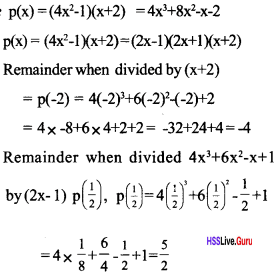### Polynomials SCERT Questions & Answers

Question 12.
Write the second-degree polynomial p(x)= x2 + x – 6 as the product of first-degree polynomials. Find also the solution of the equation p (x)=0 [Score: 4, Time: 7 minute]Question 13.
For what values of x, the polynomial 2x2 – 7x – 15 is equal to zero? Write this polynomial as the product of two first degree polynomials. [Score: 4, Time: 7 minute]Question 14.
Write the polynomial p(x) = x2 + 4x + 1 as the product of two first degree polynomials. Find the solution of the equation p (x) = 0. [Score: 4, Time: 5 minute]
x2 + 4x + 1 = (x – a) (x – b) = x2 – (a + b) x + ab(1)
a + b = –4, ab = 1, a – b = 2√3
a = –2 + √3, b = –2 – √3 (1)
x2 + 4x + 1 =(x + 2+ √3 ) (x + 2 – √3 ) (1)
x2 + 4x + 1 = 0 =>(x + 2 + √3 )(x + 2 –√3 ) = 0 (1)
x = –2 – √3 ,or x = –2 + √3

Question 15.
In the polynomial p (x) = x2+ ax + b p (3 + √2 )= 0, p (3 – √2 ) = 0, write this polynomial after finding a and b. [ Score: 4, Time: 5 minute]
p (x) = x2 + ax + b
p(3 + √2) = 0, (x – 3 – √2) is a factor (1)
p(3 – √2 ) = 0, ( x – 3 + √2) is a factor (1)
p(x) = x2 + ax + b = (x – 3 – √2) (x – 3 + √2)
= (x – 3)2 – ( √2)2 (1)
x2 + ax + b = x2 – 6x + 7 (1)

Question 16.
What number should be added to the polynomial p(x) = x2 + x – 1, so that (x – 2)is a factor of the new polynomial. [Score: 4, Time: 6 minute]
p (x) = x2 + x – 1, remainder p(2) (1)
p (2) = (2)2 + 2 – 1 = 5 (1)
For x – 2 to become a factor of p (2) must be equal to zero.
For p (2) = 0 here we have to substract 5 ffomp(x). (1)
That is, add –5 to p(x) for (x – 2) become a factor. (1)

Question 17.
What is the smallest natural number k, for which the polynomial 2x2 + kx + 6 can be written as a product of two first degree polynomials? Write down the polynomial using k and express it as the product of two first degree polynomials [Score: 4, Time: 8 minutes]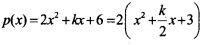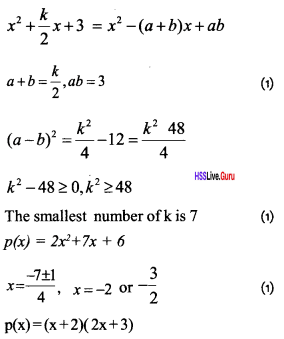Question 18.
Method to check whether(x – a), and (x + a)are factors of a polynomial P(x).
Check whether (x + 2) and (x – 5) are factors of the polynomial p(x) = x2 + 7x + 10 [Score: 4, Time: 6 minute]
When a polynomial p(x) is divided by (x – a), if p(a) = 0 then (x – a) is a factor of p(x). When a polynomial p(x) is divided by (x + a), if p(–a) = 0 then (x + a) is a factor of p(x).
p(x) = x2 + 7x + 10
p(–2) = 4 – 14 + 10 = 0 (1)
∴ x + 2 is a factor (1)
Remainder p(5) = (5)2 + 7(5) +10 (1)
= 25 + 35 + 10 ≠ 0 (1)
∴ x – 5 is not a factor

Question 19.
When dividing x2 + ax + b by (x – 2) and (x – 3) the remainder is zero. What are the numbers a and b. [Score: 3, Time: 5 minute]
p(x) = x2 + ax + b = (x – 3)(x – 2)
= x2 – 5x + 6
a = –5, b = 6 (3)

### Polynomials Exam Oriented Questions & Answers

Short Answer Type Questions (Score 2)

Question 20.
Check whether x – 1 is a factor of 3x3 – 2x2 – 3x + 2.
P(1)=3 x 13 – 2 x 12 – 3 x 1 + 2 = 0
Therefore x – 1 is a factor.

Question 21.
If (x – 1) is to be a factor of p(x) = a2x2 – 4ax + 4a – 1. What should be the value of ‘a’ ?
p(1) = 0
a2 – 4a + 4a – 1 = 0
a2 – 1 =0
a = +1 or –1

Question 22.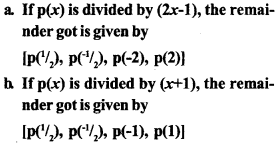Question 23.
Prove that (x – 1) is a factor of x13 – 1
p(x) = x13 – 1
p(1)= 113 – 1 = 1 – 1=0
p(1) = 0
(x – 1) is a factor of p(x)

Question 24.
Write the solution of polynomials p(x) = x2 – 7x + 12.
x2 – 7x + 12 = (x – 4)(x – 3)
p(x) = 0 (x – 4) ( x – 3) = 0
x = 4, x = 3

Question 25.
The quotient is x2 – 5x + 6 when the polynomial p(x) is divided by (x – 1), and the remainder is 7. Then
a. p(x) = (………. ) ( ……… ) + 7. Complete it
b. Find P(2).
a. p(x) = (x2 – 5x + 6) (x – 1) + 7
b. p(2) = 4 – 10 + 6 + 7 = 7

Short Answer Type Questions (Score 3)

Question 26.
a. Find the remainder when
x3 – 4x3 + 12x – 45 is divided by (x – 2)?
b. Find the value of k if the remainder is zero on dividing 2x3 + 4x2 – 10x + k by (x – 1)
a. p(x) = x3 – 4x2 – 12x – 45
remainder = p(2) = 23 – 4(2)2 + 12 x 2 – 45 = 32 – 61 = –29
b. p(x) = 2x3 + 4x2 – 10x + k; p(l) = 0
=> 2 x 13 + 4 x 12 – 10 x 1 + k = 0
2 + 4 – 10 + k = 0 – 4 + k = 0; k = 4Question 27.
Factorise 3x2 + 5x + 2 completely.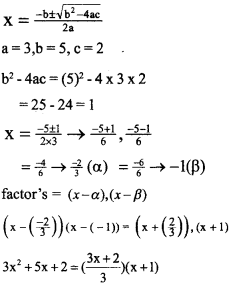Question 28.
Which first-degree polynomial is added to the polynomial 5x3 + 3x2 to get x2 – 1 as a factor.
p(x) = 5x3 +3x2 + ax + b
∴ x2 – 1 isafoctorthen p(1), p(–1) will be zero.
p(1) = 5 x 1 + 3 x 1 + a x 1 + b = 0
= 5x – 1 + 3 x 1 + ax – 1 + b = 0
a + b = –8 (1)
= 5x – 1 + 3 x 1 + ax – 1 + b = 0
–a + b = 2 (2)
Find the solutions of the equation
b = –3, a = –5 added polynomial = –5x – 3

Long Answer Type Questions (Score 4)

Question 29.
a. Find the value of k if remainder when 5x3 + 4x – 11x + k is divided by (x – 1) is 0.
b. When x3 – 2x2 + kx + 7 is divided by (x – 4) remainder is 11. Find k.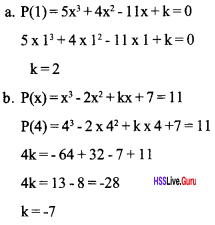Question 30.
a Show that the polynomial x + x + 1 has no first degree factors,
b. What is the remainder when the polynomial (x – 1) (x – 2) (x – 3) is divided by (x – 1)?
c. When (x – 1) (x – 2) (x – 3) + 2x + k is divided by (x – 1), the remainder is 10.
Then find out the remainder when it is divided by (x – 2).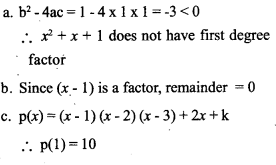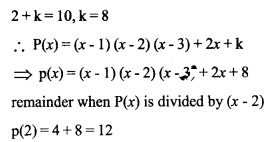Long Answer Type Questions (Score 5)

Question 31.
Write 2x2 + 5x + 3 as a product of two first degree polynomials.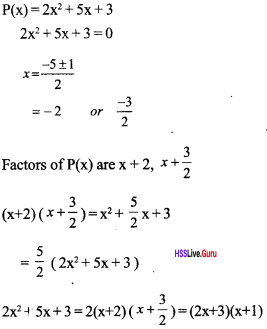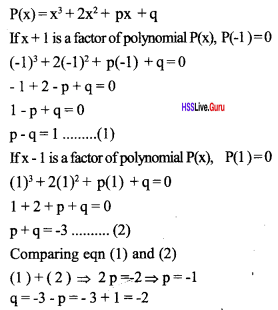.